# Orbifold notation

﻿
Orbifold notation

In geometry, orbifold notation (or orbifold signature) is a system, invented by William Thurston and popularized by the mathematician John Conway, for representing types of symmetry groups in two-dimensional spaces of constant curvature. The advantage of the notation is that it describes these groups in a way which indicates many of the groups' properties: in particular, it describes the orbifold obtained by taking the quotient of Euclidean space by the group under consideration.

Groups representable in this notation include the point groups on the sphere (S2), the frieze groups and wallpaper groups of the Euclidean plane (E2), and their analogues on the hyperbolic plane (H2).

## Definition of the notation

The following types of Euclidean transformation can occur in a group described by orbifold notation:

• reflection through a line (or plane)
• translation by a vector
• rotation of finite order around a point
• infinite rotation around a line in 3-space
• glide-reflection, i.e. reflection followed by translation

All translations which occur are assumed to form a discrete subgroup of the group symmetries being described.

Each group is denoted in orbifold notation by a finite string made up from the following symbols:

• positive integers$1,2,3,\dots$
• the infinity symbol,$\infty$
• the asterisk, *
• the symbol o, which is called a wonder (Older documents show this as a solid circle)
• the symbol x, which is called a miracle (Older documents show this as an open circle)

A string written in boldface represents a group of symmetries of Euclidean 3-space. A string not written in boldface represents a group of symmetries of the Euclidean plane, which is assumed to contain two independent translations.

Each symbol corresponds to a distinct transformation:

• an integer n to the left of an asterisk indicates a rotation of order n around a point
• an integer n to the right of an asterisk indicates a transformation of order 2n which rotates around a point and reflects through a line (or plane)
• an x indicates a glide reflection
• the symbol$\infty$ indicates infinite rotational symmetry around a line; it can only occur for bold face groups. By abuse of language, we might say that such a group is a subgroup of symmetries of the Euclidean plane with only one independent translation. The frieze groups occur in this way.
• the exceptional symbol o indicates that there are precisely two linearly independent translations.

## Chirality and achirality

An object is chiral if its symmetry group contains no reflections; otherwise it is called achiral. The corresponding orbifold is orientable in the chiral case and non-orientable otherwise.

## The Euler characteristic and the order

The Euler characteristic of an orbifold can be read from its Conway symbol, as follows. Each feature has a value:

• n without or before an asterisk counts as$\frac{n-1}{n}$
• n after an asterisk counts as$\frac{n-1}{2 n}$
• asterisk and x count as 1
• o counts as 2

Subtracting the sum of these values from 2 gives the Euler characteristic.

If the sum of the feature values is 2, the order is infinite, i.e., the notation represents a wallpaper group or a frieze group. Indeed, Conway's "Magic Theorem" indicates that the 17 wallpaper groups are exactly those with the sum of the feature values equal to 2. Otherwise, the order is 2 divided by the Euler characteristic.

## Equal groups

The following groups are isomorphic:

• 1* and *11
• 22 and 221
• *22 and *221
• 2* and 2*1

This is because 1-fold rotation is the "empty" rotation.

## Other objects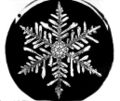A perfect snowflake would have *66 symmetry,The pentagon has symmetry *55, the whole image with arrows 55.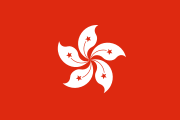The Flag of Hong Kong has 5 fold rotation symmetry, 55.

The symmetry of a 2D object without translational symmetry can be described by the 3D symmetry type by adding a third dimension to the object which does not add or spoil symmetry. For example, for a 2D image we can consider a piece of carton with that image displayed on one side; the shape of the carton should be such that it does not spoil the symmetry, or it can be imagined to be infinite. Thus we have nn and *nn.

Similarly, a 1D image can be drawn horizontally on a piece of carton, with a provision to avoid additional symmetry with respect to the line of the image, e.g. by drawing a horizontal bar under the image. Thus the discrete symmetry groups in one dimension are 11, *11,$\infty\infty$ and *$\infty\infty$.

Another way of constructing a 3D object from a 1D or 2D object for describing the symmetry is taking the Cartesian product of the object and an asymmetric 2D or 1D object, respectively.

## Correspondence tables

### Spherical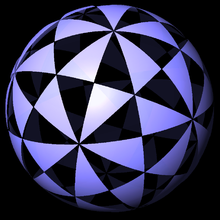532 symmetry

Spherical Symmetry Groups: (n=3,4,..)
Orbifold
Signature
Coxeter Schönflies Hermann–Mauguin Order
Polyhedral groups
*532 [3,5] Ih 53m 120
532 [3,5]+ I 532 60
*432 [3,4] Oh m3m 48
432 [3,4]+ O 432 24
*332 [3,3] Td 43m 24
3*2 [3+,4] Th m3 24
332 [3,3]+ T 23 12
Dihedral and cyclic groups: n=3,4,5...
*22n [2,n] Dnh n/mmm or 2nm2 4n
2*n [2+,2n] Dnd 2n2m or nm 4n
22n [2,n]+ Dn n2 2n
*nn [n] Cnv nm 2n
n* [2,n+] Cnh n/m or 2n 2n
nx [2+,2n+] S2n 2n or n 2n
nn [n]+ Cn n n
Special cases
*222 [2,2] D2h 2/mmm or 22m2 8
2*2 [2+,4] D2d 222m or 2m 8
222 [2,2]+ D2 22 4
*22  C2v 2m 4
2* [2,2+] C2h 2/m or 22 4
2x [2+,4+] S4 22 or 2 4
22 + C2 2 2
*221 [1,2] D1h 1/mmm or 21m2 4
2*1 [2+,2] D1d 212m or 1m 4
221 [1,2]+ D1 12 2
*11 [ ] C1v 1m 2
1* [2,1+] C1h 1/m or 21 2
1x [2+,2+] S2 21 or 1 2
11 [ ]+ C1 1 1

### Euclidean plane

#### Frieze groups

Frieze groups
Notations Description Examples
IUC Orbifold Coxeter Schönflies*
p1 ∞∞ [∞,1]+ C (hop): Translations only. This group is singly generated, with a generator being a translation by the smallest distance over which the pattern is periodic. Abstract group: Z, the group of integers under addition.p11g ∞x [∞+,2+] S (step): Glide-reflections and translations. This group is generated by a glide reflection, with translations being obtained by combining two glide reflections. Abstract group: Z
p11m ∞* [∞+,2] C∞h (jump): Translations, the reflection in the horizontal axis and glide reflections. This group is generated by a translation and the reflection in the horizontal axis. Abstract group: Z × Z2
p1m1 *∞∞ [∞,1] C∞v (sidle): Translations and reflections across certain vertical lines. The group is the same as the non-trivial group in the one-dimensional case; it is generated by a translation and a reflection in the vertical axis. The elements in this group correspond to isometries (or equivalently, bijective affine transformations) of the set of integers, and so it is isomorphic to a semidirect product of the integers with Z2. Abstract group: Dih, the infinite dihedral group.
p2 22∞ [∞,2]+ D (spinning hop): Translations and 180° rotations. The group is generated by a translation and a 180° rotation. Abstract group: Dih
p2mg 2*∞ [∞,2+] D∞d (spinning sidle): Reflections across certain vertical lines, glide reflections, translations and rotations. The translations here arise from the glide reflections, so this group is generated by a glide reflection and either a rotation or a vertical reflection. Abstract group: Abstract group: Dih
p2mm *22∞ [∞,2] D∞h (spinning jump): Translations, glide reflections, reflections in both axes and 180° rotations. This group is the "largest" frieze group and requires three generators, with one generating set consisting of a translation, the reflection in the horizontal axis and a reflection across a vertical axis. Abstract group: Dih × Z2
*Schönflies's point group notation is extended here as infinite cases of the equivalent dihedral points symmetries

#### Wallpaper groups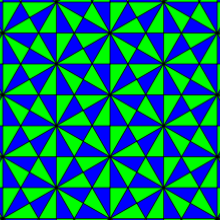632 symmetry
17 wallpaper groups
Orbifold
Signature
Coxeter Hermann–Mauguin Speiser
Niggli
Polya
Guggenhein
Fejes Toth
*632 [6,3] p6m C(I)6v D6 W16
632 [6,3]+ p6 C(I)6 C6 W6
*442 [4,4] p4m C(I)4 D*4 W14
4*2 [4+,4] p4g CII4v Do4 W24
442 [4,4]+ p4 C(I)4 C4 W4
*333 [3] p3m1 CII3v D*3 W13
3*3 [6,3+] p31m CI3v Do4 W23
333 [3]+ p3 CI3 C3 W3
*2222 [∞,2,∞] pmm CI2v D2kkkk W22
2*22 [∞,2+,∞] cmm CIV2v D2kgkg W12
22* [(∞,2)+,∞] pmg CIII2v D2kkgg W32
22x [∞+,2+,∞+] pgg CII2v D2gggg W42
2222 [∞,2,∞]+ p2 C(I)2 C2 W2
** [∞,2,∞+] pm CIs D1kk W21
*x [∞,2+,∞+] cm CIIIs D1kg W11
xx [(∞,2)+,∞+] pg CII2 D1gg W31
o [∞+,2,∞+] p1 C(I)1 C1 W1

### Hyperbolic plane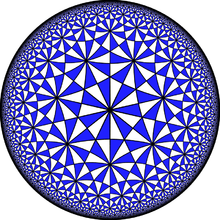732 symmetry

A first few hyperbolic groups, ordered by their orbifold characteristic are:

Hyperbolic Symmetry Groups
(-1/char) Orbifolds Coxeter
(84) *237 [7,3]
(48) *238 [8,3]
(42) 237 [7,3]+
(40) *245 [5,4]
(24) *2.3.12, *246, *334, 3*4, 238 [12,3], [6,4], [(4,3,3)], [8,3]+
(20) *2.3.15, *255, 5*2, 245 [15,3], [5,5], [5+,4], [5,4]+
(18+2/3) *247 [7,4]
(18) *2.3.18, 239 [18,3], [9,3]+
(16) *2.3.24, *248 [24,3], [8,4]
(15) *2.3.30, *256, *335, 3*5, 2.3.10 [30,3], [6,5], [(5,3,3)], [3+,10], [10,3]+
(14+2/5) *2.3.36, *249 [36,3], [9,4]
(13+1/3) *2.3.60, *2.4.10 [60,3], [10,4]
(13+1/5) *2.3.66, 2.3.11 [66,3], [11,3]+
(12+8/11) *2.3.105, *257 [105,3], [7,5]
(12+4/7) *2.3.132, *2.4.11 ... *23∞, *2.4.12, *266, 6*2 [132,3], [11,4], ..., [∞,3], [12,4], [6,6], [6+,4]
(12) *336, 3*6, *344, 4*3, *2223, 2*23, 2.3.12, 246, 334 [(6,3,3)], [3+,12], [(4,4,3)], [4+,6], ... [12,3]+, [6,4]+ [(4,3,3)]+
...

• Fibrifold notation - an extension of orbifold notation for 3d space groups

Wikimedia Foundation. 2010.

### Look at other dictionaries:

• Orbifold — This terminology should not be blamed on me. It was obtained by a democratic process in my course of 1976 77. An orbifold is something with many folds; unfortunately, the word “manifold” already has a different definition. I tried “foldamani”,… …   Wikipedia

• Ebene kristallografische Gruppe — Die ebenen kristallografischen Gruppen sind die Symmetriegruppen von periodischen Mustern oder Parkettierungen der euklidischen Ebene. Im zweidimensionalen Raum gibt es siebzehn verschiedene kristallographische Raumgruppen. Im Sinne der… …   Deutsch Wikipedia

• Ebene kristallographische Gruppe — Die ebenen kristallographischen Gruppen sind die Symmetriegruppen von periodischen Mustern oder Parkettierungen der euklidischen Ebene. Es gibt genau 17 solche Gruppen. Ihnen entsprechen im dreidimensionalen Raum die 230 kristallographischen… …   Deutsch Wikipedia

• Ornamentgruppe — Die ebenen kristallografischen Gruppen sind die Symmetriegruppen von periodischen Mustern oder Parkettierungen der euklidischen Ebene. Im zweidimensionalen Raum gibt es siebzehn verschiedene kristallographische Raumgruppen. Im Sinne der… …   Deutsch Wikipedia

• Space group — In mathematics and geometry, a space group is a symmetry group, usually for three dimensions, that divides space into discrete repeatable domains. In three dimensions, there are 219 unique types, or counted as 230 if chiral copies are considered… …   Wikipedia

• Wallpaper group — A wallpaper group (or plane symmetry group or plane crystallographic group) is a mathematical classification of a two dimensional repetitive pattern, based on the symmetries in the pattern. Such patterns occur frequently in architecture and… …   Wikipedia

• Point groups in three dimensions — In geometry, a point group in three dimensions is an isometry group in three dimensions that leaves the origin fixed, or correspondingly, an isometry group of a sphere. It is a subgroup of the orthogonal group O(3), the group of all isometries… …   Wikipedia

• Frieze group — A frieze group is a mathematical concept to classify designs on two dimensional surfaces which are repetitive in one direction, based on the symmetries in the pattern. Such patterns occur frequently in architecture and decorative art. The… …   Wikipedia

• List of mathematics articles (O) — NOTOC O O minimal theory O Nan group O(n) Obelus Oberwolfach Prize Object of the mind Object theory Oblate spheroid Oblate spheroidal coordinates Oblique projection Oblique reflection Observability Observability Gramian Observable subgroup… …   Wikipedia

• List of planar symmetry groups — This article summarizes the classes of discrete planar symmetry groups: #2 infinite set of point groups #7 Frieze groups #17 wallpaper groups Point groups There are two classes of point groups, rotational and reflectional.Point groups: Maximum… …   Wikipedia